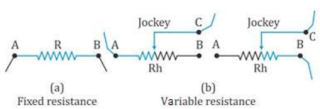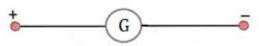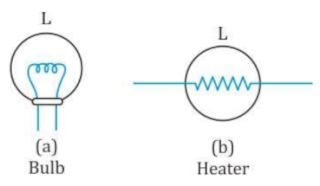# ICSE Revision Notes for Current Electricity Class 9 Physics

 Chapter Name Current Electricity Topics Covered Source of CurrentElectric CurrentElectric CircuitFlow of ElectronsUse of Energy Related Study

### Current Electricity

Sources of Direct Current

• The regular pencil cells used in daily life provide a direct current.
• Direct current is a current of constant magnitude flowing in one direction.

Kinds of Cells

 Primary Cell Secondary Cell Chemical reaction is irreversible. Chemical reaction is reversible. Chemical energy is converted into electrical energy. Electrical energy changes to chemical energy when current is passed in it, while chemical energy changes to electrical energy when current is drawn from it. It cannot be recharged. It can be recharged. It is capable of providing weak current only. It can provide small and large currents. It is light and cheap. It is heavy and costly. Examples: Simple voltaic cell, Daniel cell Examples: Lead accumulator, Ni–Fe accumulator

### Electric Current

Current (I) = Charge (Q)/Time(t)

• It is a scalar quantity. Its SI unit is ampere (A).
• Conventionally, the direction of current is taken in the direction of flow of positive charge. This direction is opposite to the direction of flow of electrons.

Symbols used in Circuit Diagrams

A circuit has various electric components:

1. Sources of current: Current is of two types − alternating (or AC) and direct (or DC). AC is obtained from the mains in our house or an AC generator, while DC is obtained from a cell or battery.

2. Key: It is used to put the current on and off in a circuit.

When a key is closed, i.e. the plug is inserted, the circuit is called a closed circuit

3. Resistance wire, resistance box, rheostat or variable resistance: A resistance wire is made up of an alloy of nichrome and has a fixed resistance value depending on its length and thickness. A resistance box is provided with several standard resistances connected in series. A rheostat is a continuous varying resistance device used to adjust the magnitude of current in a circuit. Fixed resistance is represented by the symbol R, and the rheostat or variable resistance is given by the symbol Rh.4. Ammeter: An ammeter is used to measure the magnitude of current flowing in a circuit. It is to be connected in series.5. Voltmeter: A voltmeter is used to measure the magnitude of potential difference between two points in a circuit. It is to be connected across two points, i.e. in parallel to the flow of current.5. Galvanometer: A galvanometer is used in a circuit when a small current is to be measured or to know the direction of flow of current. It is also connected in series in the circuit.6. Load: An appliance connected in a circuit is called load. It is represented by the symbol L.7. Connecting wires: The wires used to connect different electrical components are called connecting wires. They are made of highly conducting metal such as copper or aluminium.

### Simple Electric circuit

Functions of Various Components in a Circuit

• The key completes the circuit. When it is open, the bulb does not glow, and the ammeter and voltmeter do not show any reading.
• When the key is closed, the bulb starts glowing, and the ammeter and voltmeter show some reading.
• When the resistance is varied by sliding the jockey of the rheostat, the glow of the bulb changes and so do the readings on the ammeter and voltmeter.

Insulators and Conductors

1. Insulators: The substances which do not allow the current to flow through them are called insulators. They do not have free electrons and they offer very high resistance.
2. Conductors: The substances which allow the current to flow through them easily are called conductors. They have a large number of free electrons and they offer very less resistance.

### Flow of Electrons

• When a positively charged conductor is joined by a metal wire to an uncharged conductor, the electrons flow from the uncharged conductor to the positively charged one.
• When a negatively charged conductor is joined by a metal wire to an uncharged conductor, the electrons flow from the negatively charged conductor to the uncharged one.
• When a positively charged conductor is joined by a metal wire to a negatively charged conductor, the electrons flow from the negatively charged conductor to the positively charged one.

Direction of Flow of Current: Conventional and Electronic

Potential Difference

• Potential difference between two conductors is equal to the work done in transferring a unit positive charge from one conductor to the other through the metallic wire.
V = W/q
• It is a scalar quantity. Its SI unit is volt (V).
• The potential difference between two points is said to be 1 volt if the work done in transferring 1 coulomb of charge from one point to the other is 1 joule.

Electrical Resistance

• There is always some obstruction in the current which flows through a conductor such as a metal wire, and this obstruction is called its electrical resistance
• According to Ohm’s law, if current I flows through a wire when potential difference across the ends of a wire is V, then the resistance offered by the wire is
Resistance (R) = Potential difference across the wire (V)/Current flowing in the wire (l)

Factors on which the Resistance of a Conductor Wire Depends

1. Length of the wire: Resistance (R) ∝ length l
2. Area of cross-section of the wire: Resistance (R) ∝ 1/Area of cross-section (A)
3. Material of the wire: The resistance depends on the material of the wire as there is different concentration and different arrangement of atoms in different materials.
4. 4. Temperature of the wire: A higher temperature of the wire causes the ions in it to vibrate more rapidly. As a result, the number of collisions increases, and hence, the resistance increases.

### Efficient Use of Energy

Efficient use of energy means to reduce the cost and amount of energy to be used to provide us the various products and services.

Examples:

• The use of compact fluorescent lights (CFL’s) saves up to 67% energy, and they may last 6–10 times longer than the regular incandescent bulbs.
• Insulating a home allows a building to use less heating and cooling energy to achieve and maintain a comfortable temperature. This will reduce the cost of heating devices in winter and cooling devices in summer.
• Modern energy-efficient appliances such as refrigerators, ACs, ovens, dryers, freezers, stoves, dishwashers, etc. use less energy than the older ones. Nowadays appliances are star rated according to their efficiency in using electricity.
• The fuel efficiency in a vehicle can be increased by reducing the weight of the vehicle by using advanced tyres and computer-controlled engines.
• A building’s location and its surroundings play a key role in regulating its temperature and illumination. Proper placement of windows and use of features which reflect light into a building can reduce the need for artificial light.
• The use of fluorescent lights or natural skylight instead of the traditional incandescent light bulbs reduces the amount of energy required to attain the same level of illumination.
• The use of advanced boilers and furnaces in industry can save sufficient amount of energy in attaining high temperature by burning less fuel.